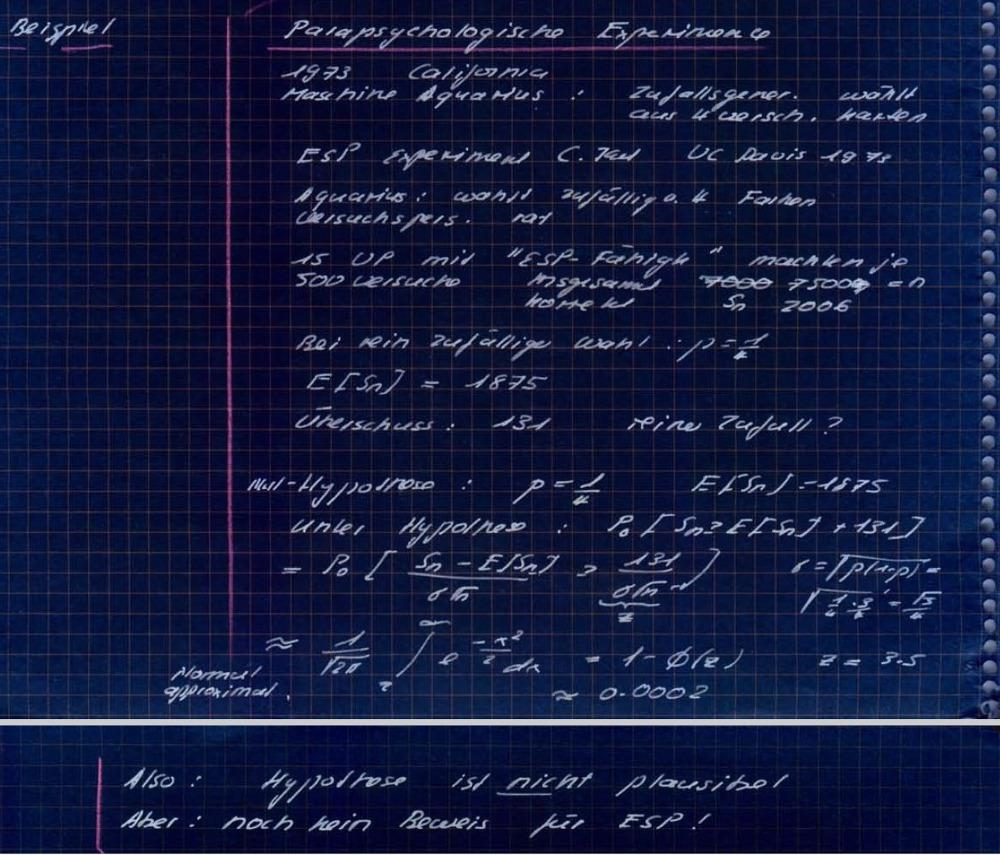# ESP amusement

An article "Feeling the Future: Experimental Evidence of Bem for Anomalous Retroactive Influences on Cognition and Affect" will appear in the "Journal of Personality and Social Psychology". A NYT article covers this. The paper stirred discussion about the peer review process which led to the publication. We will learn a bit of probability in this course to understand this better:

I have still my notes from a great undergraduate course in statistics I took from Hans Foellmer. Foellmer reported in one of his classes about an ESP experiment from 1973 and explains in that example the reasoning with which one can reject a Null hypothesis. It is completely analog to the experiments done by Bem but simpler to explain since it involves only to guess the right color from 4 colors:

n = 7500 experiments were done to guess from 4 random colors. There were 2006 correct. One would expect E[Sn] = 1875. The question is whether the 131 surplus is statistically significant. Under the Null-hypothesis { p = 1/4 } one has a standard deviation of sigma = sqrt(p(1-p) = sqrt(3)/4 = 0.43301 and z= 131/sigma sqrt(n) = 3.5. Under the null hypothesis assumption and the assumption that data are normally distributed, the probability that the experiment gives a value larger or equal than 131 is:
```  P0[ Sn= E[Sn] + 131] = P0[ (Sn-E[Sn)/sigma sqrt(n) > z ]
```
The later value is looked up with Mathematica:
```z=3.5; Integrate[ (1/Sqrt[2Pi]) Exp[-x^2/2],{x,z,Infinity}]
```
and is about 0.0002, suggesting that the Null Hypothesis is not plausible. However, it is a pitfall to induce from this that the null hypothesis is false. In a rebuttal, Rouder and Morey use a Bayes factor analysis. The Bayes factor is defined as
```B = P[data|H1]/P[data|H0]
```
where H0 is the null hypothesis and H1 the complement. In Foellmer's schoolbook example B= ~ 5000. This looks like a lot of evidence for the data. But since the Null hypothesis has a priory very low probability, the posteriority odds
```P[ H1 | data]/P[ H0 | data ]   = B  P[H1]/P[H0]
```
is much smaller. The apriory odds P[H1]/P[H0] are now very low: thousands of other experiments so far have shown that ESP does not work. In a situation like ESP, one has to assume an apriory odd of a million at least.

 An ESP experiment proving that ESP works, must have a Bayes factor much larger than 5000.

Here is scan of my own class notes, I had copied from the blackboard, when I was an undergraduate: (You see I'm a data hog and do not forget or throw anything!)